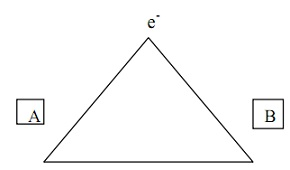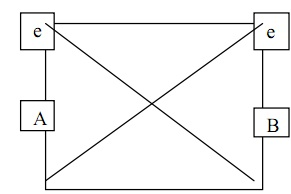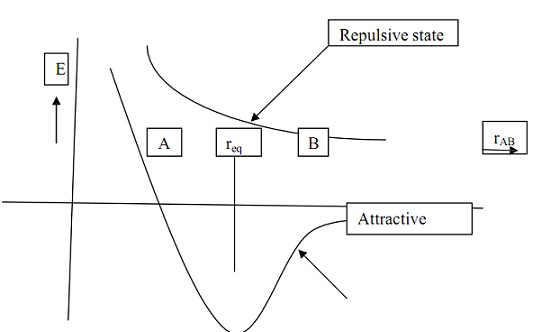#### Application of Schrodinger Wave Equation, Chemistry tutorial

Introduction

Chemical bonding includes two or more atoms coming mutually due to the force of interaction between them. The aggregate molecule that is shaped has lower energy than the divided fragments and when this energy lowering exceeds 41.8 kJ mol-1of atoms, we say that a chemical bond exists

Definition of Bond Formation in Molecules

If we think 2 atoms A and B divided via several distance r. depending on the magnitude of r, there is bound to be attraction or repulsion. If the attractive force is greater than the repulsive force, the net consequence is a chemical bond being formed whereas the converse is as well true.

The wave function

For a scientist, knowing that matter acts as a wave is helpful only if one recognizes something about that wave. The wave function is a mathematical function describing the wave. For instance, y(x) = A sin(kx) might be the wave-function for a one-dimensional wave that exists along the x-axis. Matter waves are three-dimensional; the appropriate wave function depends on the x, y, and z coordinates of the system being studied (and sometimes on time as well). We conventionally label the wave function for a 3-dimensional object as ψ(x, y, z). In the year 1926 Erwin Schrödinger introduced a mathematical equation whereby, if one recognizes the potential energy acting on an object, one can compute the wave function for that object. Heisenberg had previously introduced a mathematical formalism for performing quantum mechanics computations, with no explicitly including the idea of waves. It was later exposed that, even though the approaches of Schrödinger and Heisenberg looked extremely different, they made accurately the similar predictions. In practice, the Schrödinger formalism is more helpful for illumination the problem being studied, and the Heisenberg methodology permits for more straightforward computation. Accordingly, a mixture of the two approaches is typically utilized in modern quantum chemistry. Once we know the wave function of the atom or molecule under study, we can compute the properties of that atom or molecule.

Quantum Mechanics of Atoms

A precise solution for Schrödinger's wave equation can be attained for the hydrogen atom; though, for larger atoms and molecules (that enclose more than one electron), Schrödinger's equation can be solved only approximately. Even though this might sound as restrictive as to make the equation useless, there are well-established approaches that permit for practical and accurate calculations on atoms and molecules. This is done by making some assumptions about larger systems depend upon the hydrogen atom, as described below.

When the Schrödinger equation is solved for the hydrogen atom, the consequential wave functions for the different possible energies that the atom can have are utilized to find out atomic orbitals. An orbital is a region in space where an electron is most probable to be found.  For instance, the lowest-energy wave function for a hydrogen atom is the so-termed 1s orbital, which is a spherical region in space surrounding the nucleus. For some higher-energy states, the orbitals are not necessarily spherical in shape (for example, the 2p orbital).

For atoms larger than hydrogen, one assumes that the orbitals occupied via the electrons have the similar shape as the hydrogen orbitals but are different in size and energy. The energies corresponding to such orbitals might be found through solving an approximate version of Schrödinger's equation. Such atomic orbitals, in turn, might be utilized as the building blocks to the electronic behavior in molecules, as we shall see below. As it happens, two electrons may share an atomic orbital; we say that these electrons are paired. Chemists have developed a system of rules for computing which orbitals are occupied in that atom; calculations can then be done to determine the energies of the electrons in the atoms.

Quantum Mechanics of Molecules

Molecules are held together by covalent bonds. The simplest description of a covalent bond is a shared pair of electrons. There are two basic approaches to modeling covalent bonds in molecules: the valence bond model and the molecular orbital model. In the valence bond model, we think of atomic orbitals on each of 2 atoms combining to form a bond orbital, with one electron from each atom occupying this orbital. Both the bond orbital and the electron pair now "belong" to both of the atoms. This sharing of electrons carries about a lowering in the energy, which makes the formation of molecules from atoms an energetically favorable process. The valence bond model gives the simplest quantum mechanical picture of chemical bonding, but it is not the best method for accurate calculations on molecules containing more than two atoms.

Molecular orbital theory differs from valence bond theory in that it doesn't need the electrons involved in a bond to be localized between two of the atoms in a molecule.  Instead, the electron occupies a molecular orbital, which might be spread out over the entire molecule. As in the valence bond approach, the molecular orbital is shaped by adding up contributions from the atomic orbitals on the atoms that make up the molecule. This approach, which doesn't explicitly model bonds as existing between two atoms, is somewhat less appealing to the intuition than the valence bond approach.

Though, molecular orbital computations classically yield better predictions of molecular structure and properties than valence bond techniques. Accordingly, most commercially available quantum chemistry software packages rely on molecular orbital process to perform computations.

The Hydrogen Molecule ion

The hydrogen molecule ion consists of 2 protons and 1 electron. It is consequently the simplest molecular system that can be encountered in nature. The molecule ion is frequently symbolized asFig: The Hydrogen Molecule ion

The hydrogen molecule ion is represented by the triangle above. The 2 hydrogen atoms are symbolized via A and B while the only electron is represented by e-. The distance between A and B is the internuclear distance rAB while the distance between the electron and each of the atoms refers to the sides of the triangle, rA and rB, correspondingly.

The interactions among the species are as follow:

1. Attractive interaction between the electron and nucleus A

2. Attractive interaction between the electron and nucleus B

3. Repulsive interaction between the two nuclei.

The potential energy (U) for the hydrogen molecule ion is

U = - e2/rA-  e2/rB + e2 /rAB

The   first  two  terms  in  equation  represent  the  electrostatic attraction between  the  nuclei  and  the  electron  while  the  last  term represents the repulsion between the nuclei. The kinetic energy (K.E.) for the molecule is

K.E = P2/2m= 1/2m(Px2 + Py2 +Pz2)

Where p= momentum of the electron,

m = mass of the electron

The electron is assumed to be moving in the three directions in space. It should be noted that we have assumed rAB fixed, which implies that only one K.E. term needs to be considered;  the K.E. due to the electron motion. The 3D Schrodinger equation is

δ2φ/ δx2 + δ2φ/ δy2 + δ2φ/ δz2 + 8 π2m/h2 [E- U)(x,y,z)]φ = 0

that can be written as

H = E

Where H = -h2 / 8 π2m (δ2/ δx2+ δ2/ δy2 + δ2/ δz2) + U (x,y,z)

Known as the Hamiltonian operator and

2 = δ2/ δx2+ δ2/ δy2 + δ2/ δz2

is a Laplacian operator.

Equation is known as the Hamiltonian form of Schrodinger equation.

It should also be noted that

H = - h2/2m * 2 + U(x,y,z)

The Schrodinger equation for the hydrogen molecule ion is

( -h2/2m  * ∇2  +e2/rAB - e2/rA- e2/rB) + Eφ

This wave equation is simple and it is possible to get an exact solution for it.

The hydrogen molecule

The hydrogen molecule has 2 electrons (e1 and e2) and two nuclei (A and B). The coordinate in the hydrogen molecule is as revealed below.Fig: The hydrogen molecule

The interactions among the species are as follows.

1. Repulsive interaction between the 2 electrons;

2. Attractive interaction between the first electron and nucleus A;

3. Attractive interaction between the first electron and nucleus B;

4. Attractive interaction between the second electron and nucleus A;

5. Attractive interaction between the second electron and nucleus B;

6. Repulsive interaction between the two nuclei.

The steps includes in writing the Schrodinger equation for this molecule are:

1. Writing the expression for the K.E.;

2. Writing expression for the P.E.;

3. Inserting the two expressions above in the Schrodinger equation.

Total K.E. for the system = (K.E.)1+(K.E.)2   =

K.E. = P12/2m +P22/2m

Where 1, 2 stand for the first and second electrons, respectively.

Total K.E =   1/2m(Px12 + Py12 + Px12 )+  2/2m ( Px22  +  Py22 + PZ22)

U(x,y,z)= -e2 /r1.A -e2/r1.B - e2/r2.A-e2/r2B + e2/rAB+e2/r12

The Schrodinger equation for the hydrogen molecule is

- [h2/2m(∇21 +∇22 ) - e2 /r1.A - e2/r1.B - e2/r2.A - e2/r2B + e2/rAB + e2/r12 ] ψ= Eψ

which transforms to

(∇21 +∇22 ) ψ + 8π2m/h2( E + e2 /r1.A + e2/r1.B + e2/r2.A+ e2/r2B - e2/rAB - e2/r12)ψ = 0

This is the needed equation.

Whereas it is possible to obtain an exact solution to Schrodinger equation for hydrogen molecule ion, by separating into any other coordinates (for example polar coordinates),  we can't solve  the  equation  for  the  hydrogen molecule  because  of  the  presence  of  repulsive  term  (e2/r12). In this situation, we utilize an estimated technique to get the solution to the Schrodinger equation. We always aim at the energy of the system in joint form compared through when the atoms are far apart. We have assumed that both nuclei A and B are fixed meaning that their K.E. will be almost zero. Thus instead of the

K. E. = (K.E.)A + (K.E)B  + (K.E.)1 +(K.E.)2

We have neglected that due to the nucleus in together equations for hydrogen molecule above. The justification for doing this is that the motions of nuclei in common molecular vibrations are so slow evaluated to the motions of the electrons that it is possible to compute the electronic states on assumption that the nuclei are held in attached position.

This is the Born-Oppenheimer estimate. What this means is that in equation for hydrogen molecule for example, we consider rAB constant. We can therefore compute E for this fixed value of rAB. If we change the value of rAB, an analogous value of E(rAB) can be got. Thus it should be possible to make a plot of E(rAB) against rAB as shown in the Figure below (Figure) which is known as the potential energy curve. In the Figure, the equilibrium inter nuclear distance is req and corresponds to the region where the total energy of the system is a minimum (that is the system is stable there). It is termed the bond length. When r is small, the molecule is unbalanced due to high repulsive force and as well whenever r is huge, it is unstable due low attractive force that might not be huge enough to offset the repulsive force. In between position A and B, in the Figure the molecule is stable but is not stable at req.Fig: Potential Energy Curve Showing the Variation of the Total Energy of the System with the Internuclear Distance rAB.

We cannot obtain exact value of E for the hydrogen molecule because of the presence of the repulsive term which made it impossible for us to separate the molecule into hydrogen atoms for which two electrons are involved. As well the occurrence of n2 and n22 in the Schrodinger equation for the hydrogen molecule suggests that we should look for another way of solving the equation. The approximate method known as the variation process is often employed.

Variation method

It is an approximate process for solving the Schrodinger equation as in equation. Since an exact solution of it isn't probable. The Schrodinger equation in Hamiltonian form is Hψ = Eψ.

Multiplying both sides by ψ* and integrating over all space to obtain

∫ ψ*H ψd τ   = ∫ ψ* E' ψd τ

Therefore E' =   ∫ ψ*H ψd τ / ∫ ψ* ψd τ

Where E' specifies the energy that consequences from an inexact wave function and H is the complete Hamiltonian for the system.  Note that E' is steady.  Equation provides a true value for E' if ψ is the precise wave function. Though, ψ is only an approximation therefore the value of E' will not be true value. In essence, we want to obtain an approximation value for E' for that acceptable wave function exists.

The latter will be acceptable if finite and single valued. It must as well be continuous and its 1st and second derivatives must be continuous as well. It should be possible to standardize the function.

In molecular terms, we don't know ψ, so we guess at a function, ψ1. From this we can compute a corresponding value for E'. We can as well guess at ψ2 and hence deduce E1'. We can also guess at ψ2 and hence E2' and so on. The variation theorem states that out of the E1', E2',,.... En', one will be the smallest and that if the true energy of the system is E, the lowest computed value of Ei' (i= 1,2,3,4,,,) isn't smaller than the true energy of the system. That is E'≥ Etrue.

Because the true energy of the system is the minimum energy from the potential energy curve, E' will represent the smallest eigen value computed. If we contain ψ1, ψ2 and ψ3, instead of doing the calculation three times, we get a linear combination of such and feed the effect into equation. Thus ψ = c1ψ1 + c2 ψ2 +c3ψ3 Ψ is a master wave function and c1, c2, c3 are recognized as the difference parameters. We shall then attain the energy E' in terms of c1, c2, c3, that we can minimise. The consequence attained will be originated to be greater than or equal to the true energy of the system.

Tutorsglobe: A way to secure high grade in your curriculum (Online Tutoring)